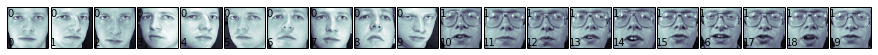# Learning Scikit-learn: Machine Learning in Python¶

## IPython Notebook for Chapter 2: Supervised Learning - Image Recognition with Support Vector Machines¶

_In this notebook, we show how to perform face recognition using Support Vector Machines. We will use the Olivetti faces dataset, included in Scikit-learn. More info at: http://scikit-learn.org/stable/datasets/olivetti_faces.html_

Start by importing numpy, scikit-learn, and pyplot, the Python libraries we will be using in this chapter. Show the versions we will be using (in case you have problems running the notebooks).

In :
%pylab inline
import IPython
import sklearn as sk
import numpy as np
import matplotlib
import matplotlib.pyplot as plt

print 'IPython version:', IPython.__version__
print 'numpy version:', np.__version__
print 'scikit-learn version:', sk.__version__
print 'matplotlib version:', matplotlib.__version__

Populating the interactive namespace from numpy and matplotlib
IPython version: 2.1.0
numpy version: 1.8.2
scikit-learn version: 0.15.1
matplotlib version: 1.3.1


Import the olivetti faces dataset

In :
from sklearn.datasets import fetch_olivetti_faces

# fetch the faces data
faces = fetch_olivetti_faces()

print faces.DESCR

Modified Olivetti faces dataset.

The original database was available from (now defunct)

http://www.uk.research.att.com/facedatabase.html

The version retrieved here comes in MATLAB format from the personal
web page of Sam Roweis:

http://www.cs.nyu.edu/~roweis/

There are ten different images of each of 40 distinct subjects. For some
subjects, the images were taken at different times, varying the lighting,
facial expressions (open / closed eyes, smiling / not smiling) and facial
details (glasses / no glasses). All the images were taken against a dark
homogeneous background with the subjects in an upright, frontal position (with
tolerance for some side movement).

The original dataset consisted of 92 x 112, while the Roweis version
consists of 64x64 images.



Let's look at the data, faces.images has 400 images of faces, each one is composed by a matrix of 64x64 pixels. faces.data has the same data but in rows of 4096 attributes instead of matrices (4096 = 64x64)

In :
print faces.keys()
print faces.images.shape
print faces.data.shape
print faces.target.shape

['images', 'data', 'target', 'DESCR']
(400, 64, 64)
(400, 4096)
(400,)


We don't have to scale attributes, because data is already normalized

In :
print np.max(faces.data)
print np.min(faces.data)
print np.mean(faces.data)

1.0
0.0
0.547046


Plot the first 20 images. We have 40 individuals with 10 different images each.

In :
def print_faces(images, target, top_n):
# set up the figure size in inches
fig = plt.figure(figsize=(12, 12))
fig.subplots_adjust(left=0, right=1, bottom=0, top=1, hspace=0.05, wspace=0.05)
for i in range(top_n):
# plot the images in a matrix of 20x20
p = fig.add_subplot(20, 20, i + 1, xticks=[], yticks=[])
p.imshow(images[i], cmap=plt.cm.bone)

# label the image with the target value
p.text(0, 14, str(target[i]))
p.text(0, 60, str(i))


In :
print_faces(faces.images, faces.target, 20)Plot all the faces in a matrix of 20x20, for each one, we'll put it target value in the top left corner and it index in the bottom left corner. It may take a few seconds.

In :
print_faces(faces.images, faces.target, 400)